Garrett Mayock's Blog

# Fixing inefficient optimization code in Python

### A brief detour from Managing Supply Chain Operations to make some inefficient code better.Garrett Mayock posted 2019-03-02 02:21:52 UTC

Quick review

So, let's review. In my last blog, I created a Holt's Trend Model in Excel and Python. Here's what the formulas looked like in the Excel file: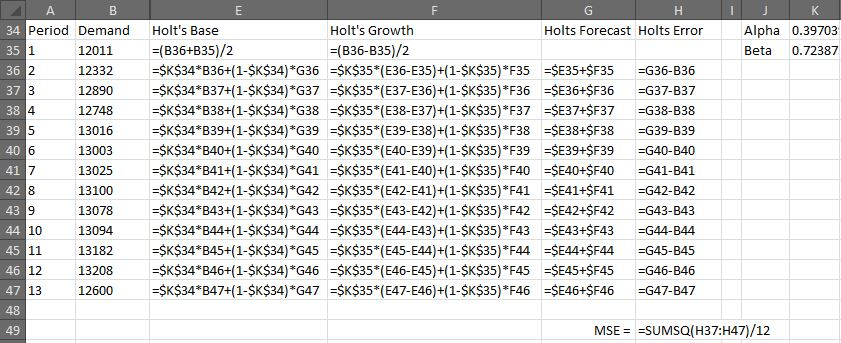Excel Solver could minimize MSE (cell H49) by optimizing α and β (cells K34 and K35) from 0.1 and 0.2 respectively, each constrained between 0 and 1, in a split second. The code I wrote in Python took nearly two minutes to run. I knew this was ridiculous, and a quick glance at the code revealed it was probably because it was hugely recursive. Today, I set out to optimize the code and see if I could match that "split second" time frame.

Exploring what went wrong in the inefficient code

Counting the loops in the inefficient code

Here is the Python code I wrote:

```# This ingests just the period and demand data, for the 13 periods

holts_base = (df['Demand']+df['Demand'])/2
holts_growth = holts_base - df['Demand']

def holtsForecast(period, alpha, beta):
holts_forecast = holtsBase(period, alpha, beta) + holtsGrowth(period, alpha, beta)
return holts_forecast

def holtsBase(period, alpha, beta):
if period == 1:
base = holts_base
return base
else:
base = alpha*df['Demand'][period-1]+(1-alpha)*holtsForecast(period-1, alpha, beta)
return base

def holtsGrowth(period, alpha, beta):
if period == 1:
growth = holts_growth
return growth
else:
growth = beta*(holtsBase(period, alpha, beta)-holtsBase(period-1, alpha, beta))+(1-beta)*holtsGrowth(period-1, alpha, beta)
return growth

def holtsMSE(x):
sse_val = 0
sse_count = -1
for i in range(1,max(df['Period'])):
sse_val = (holtsForecast(i, x, x)-df['Demand'][i])**2 + sse_val
sse_count += 1
mse_val = sse_val/sse_count
return mse_val

# This is the actual optimization
from scipy.optimize import minimize
result = minimize(holtsMSE, [0.1,0.2], bounds=((0,1),(0,1)))
print (result.x)
```

That took nearly 2 minutes to run (108.711s). A quick look at the functions I define make it pretty obvious why - it's incredibly recursive. I did a little test to see how many times the functions were called by adding a global variable named "f_call_count" and set it to start at 0 and add 1 to a running total every time a function was called. A single run of holtsMSE() caused it to run 1,195,723 times. That's once for holtsMSE(), and 220+217+213+212+211+210+29+27+26+23+21 for the other functions. Just for kicks and giggles, I ran the same function when running the optimizer: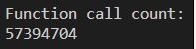Functions were called 57,394,704 times. Interestingly, we can see the optimizer ran exactly 48 times by dividing the optimization function call count by the holstMSE() call count, but either way, it's clear we have a lot of work to do.

Logging the function calls

The first thing I want to do is log what's happening. I'll set up a logger:

```# This sets up the logger
import logging.handlers
import os
handler = logging.handlers.WatchedFileHandler(os.environ.get("LOGFILE", "filepath/log/holtsoptimizer.log"))
formatter = logging.Formatter(logging.BASIC_FORMAT)
handler.setFormatter(formatter)
root = logging.getLogger()
root.setLevel(os.environ.get("LOGLEVEL", "DEBUG"))
```

And import sys:

```import sys
```

Then add code to the holtsForecast(), holtsBase(), and holtsGrowth() functions:

```logging.debug((sys._getframe().f_code.co_name,": ",period))
```

What that does it log a debug level message. The message has three parts. The first part is from the sys module, which provides access to the system parameters and functions. The next part, _getframe(), returns the frame at the top of the call stack. UC Santa Barbara wrote a great explanation of frames and call stacks here. The next part, f_code, is the code object being executed in the frame that was returned by _getframe(). What that means, is, since I put that code into each function, the f_code is the function itself. Finally, the co_name is the name with which this code object was defined, which of course means the function name. Therefore, for each function I wrote, that code snippet logs the function name, a colon, a space, and then the period being called. Furthermore, I added a similar code to the holtsMSE() function to track the parameter array when using scipy.optimize.minimize:

```logging.debug((sys._getframe().f_code.co_name,": ",x))
```

You'll see the only difference is that the variable it logs is x, not period.

With this in mind, I ran a few variations of the code while logging. After testing the logging code with a couple short runs, the first big run was the optimization function itself, which took over six minutes to run and created a 37.12 MB log file. But that's a bit excessive if I just want to visualize what's happening, so I edited the holtsMSE function for loop to run for three and four periods, leading to logs of a manageable size. I'll take a look at the three loop log and the four loop log next. (Note - I've renamed the file extension to .txt instead of .log due to server settings).

Making sense of the logs

The first thing I'll do is visualize the three and four loop logs in Excel. I copy and paste the log file into Excel, then use "Text to Columns" to split the columns on the ":" delimiter. This gets the period numbers that were logged all vertically aligned, allowing for easy visual review. Then, I use conditional formatting rules to color cells by the name of the function they contain. Then I looked at the periods to see how the size grows. Remember, the holtsMSE() function begins by calling for a forecast for period 1, then 2, and so on, until the loop ends.

Not counting the original holtsMSE() function, the forecast for a single period calls three functions once - holtsForecast, then holtsBase, and finally holtsGrowth. The forecast for two periods calls twelve more functions - for a total of 15, plus the original holtsMSE(). The forecast for three periods calls 39 more, for a total of 54+. The forecast for four periods calls 120 more, for a total of 174+. Therefore, the relationship for growth of functions when calling holtsForecast() is callst = (callst-1 + 1) * 3, or for holtsMSE() where number of periods n where t0 = 0: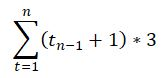A few quick visualizations I did up in Word's SmartArt (for lack of a better tool - please let me know if you know pythonic ways to create the following! I didn't want to get too deep down that detour at this juncture) help show how quickly the functions grow. For one level of forecast, it's simple: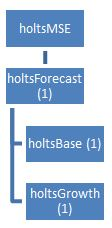With two levels of forecast, it becomes apparent that the code is not efficient: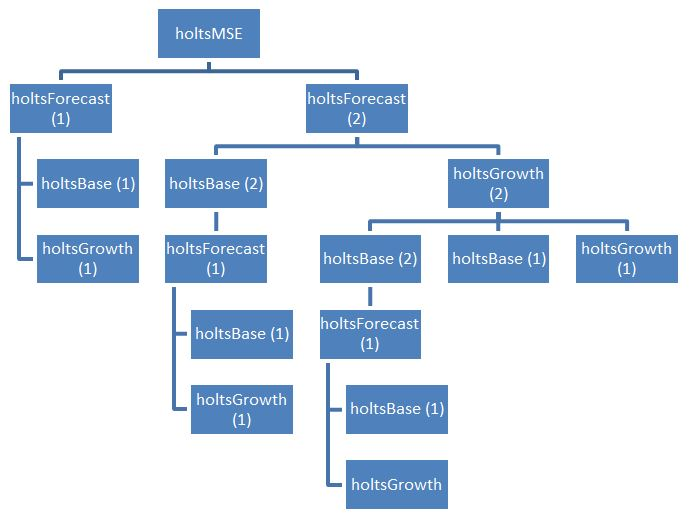With three levels of forecast we see how exponentially it balloons: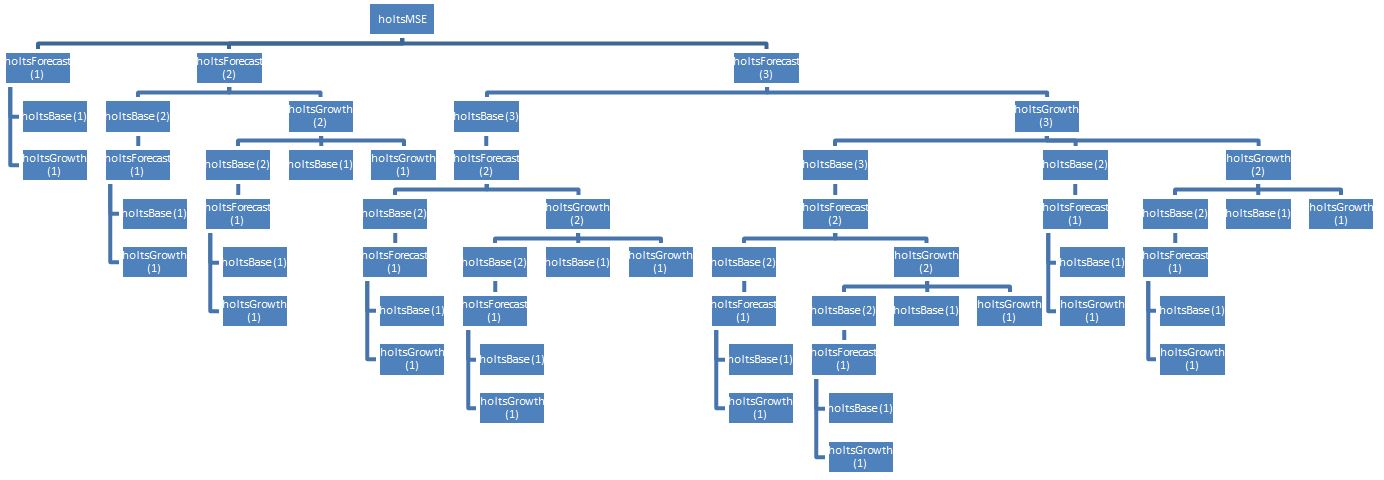Keep in mind that's only three levels. With the full 12 periods, there'd be 1,195,723 boxes on that diagram. I shudder to think of it.

Now, it's clear that the recursive function design is causing problems. Every time holtsBase() is called, it triggers a new forecast for all previous periods. holtsBase() is called by both other functions. It gets pretty loopy.

Writing better code

I think one way to do this would be to persist the information. That's essentially what Excel is doing - so that's the first thing I'll try. I begin the same way - importing the data and declaring the period 1 base and growth rate for Holt's model

```# This ingests just the period and demand data, for the 13 periods
df = pd.read_excel('D:/Keep/Learning/Supply Chain/Managing Supply Chain Operations/xL/chapter_2_instruction.xlsx', sheet_name='2.4_figure_2.15_new_exercise', header=33, index_col=None, nrows=13, usecols=[0,1])

# This sets the period 1 base level and growth rate for Holt's model
holts_base = (df['Demand']+df['Demand'])/2
holts_growth = holts_base - df['Demand']
```

Then, I'll create a function which stores the forecast results in a list within it, and returns the entire list at once:

```# This function creates a list which contains the results of Holt's model
def holtsModel(period, alpha, beta):
global holts_persisted_list
holts_persisted_list = [['Period','Holt\'s base','Holt\'s growth','Holt\'s forecast'],[1,holts_base,holts_growth,holts_base+holts_growth]]
for i in range(1, period+1):
if i == 1:
holts_persisted_list[i]
else:
current_demand = df['Demand'][i-1]
last_base = holts_persisted_list[i-1]
last_forecast = holts_persisted_list[i-1]
new_base = alpha*current_demand+((1-alpha)*last_forecast)
new_growth = beta*(new_base-last_base)+(1-beta)*holts_persisted_list[i-1]
holts_persisted_list.append([i,new_base,new_growth,new_base+new_growth])
return holts_persisted_list
```

Many things are different here than from before - there is one fundamental difference I will call out. The results are persisted. That means once a period's results are calculated, it's stored in the list. When a later period needs calculation, it simply looks at the stored results of the model from the holts_persisted_list list, rather than calculating them all over again, as the last model did. This eliminates a huge amount of the recursiveness.

The next step was to test the holtsMSE() function. I made some changes to it - rather than calling the forecasting function every time the loop runs, it simply calls it once to create the list, and then looks at the list from within the for loop:

```mse_max = len(df['Period'])

def holtsMSE(x):
global holts_persisted_list
sse_val = 0
sse_count = 0
holtsModel(mse_max, x, x)
for i in range(1,mse_max):
global holts_persisted_list
holts_forecast = holts_persisted_list[i]
current_demand = df['Demand'][i]
sse_val = (holts_forecast-current_demand)**2 + sse_val
sse_count += 1
mse_val = sse_val/sse_count
return mse_val
```

Note how I declare "mse_max". That's just a way of making the number of loops dynamic - if we had a different amount of demand information in the "df" dataframe, the holtsMSE() function's for loop range would automatically reflect that.

Again, the big difference in this function is that the function holtsModel() is only called once, again because the results are persisted. That means instead of having to call the forecast function once per period we want forecasted, we just call it once overall and refer back to the results.

This results in a great improvement in performance thus far. The algorithm (including running holtsMSE()) is completing in ~0.04 seconds, well down from the consistent 3.05 seconds in the previous code, or some 72x faster. Nice.

Let's see how much quicker it is optimizing α and β. I'll set the initial_guess for α and β back to 0.1 and 0.2 respectively, as they were testing last time, and use the same code as before:

```test_alpha = 0.1
test_beta = 0.2
initial_guess = [test_alpha, test_beta]

# This is the actual optimization
from scipy.optimize import minimize
result = minimize(holtsMSE, initial_guess, bounds=((0,1),(0,1)))
print ("\n",result.x)
```

You guys. Look: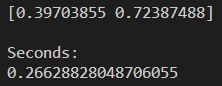408 times faster!

Well shoot I'ma call that that. Code = optimized.

Next steps

Back to the book! I'll be working on seasonal forecasting models next, and that'll finish up the chapter 2 instruction section. Two case studies follow, which I am not sure if I'll blog about, then the exercises, which I will put in a blog. I'll keep you posted!